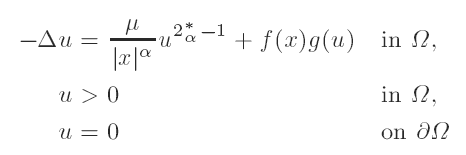Home

# Existence of positive solutions of some semilinear elliptic equations with singular coefficients

## Abstract

In this paper we consider the semilinear elliptic problem in a bounded domain Ω ⊆ Rn,where μ ≥ 0, 0 ≤ α ≤ 2, 2α* := 2(n − α)/(n − 2), f : Ω → R+ is measurable, f > 0 a.e, having a lower-order singularity than |x|-2 at the origin, and g : R → R is either linear or superlinear. For 1 < p < n, we characterize a class of singular functions Ip for which the embeddingis compact. When p = 2, α = 2, fI2 and 0 ≤ μ < (½(n − 2))2, we prove that the linear problem has-discrete spectrum. By improving the Hardy inequality we show that for f belonging to a certain subclass of I2, the first eigenvalue goes to a positive number as μ approaches (½(n − 2))2. Furthermore, when g is superlinear, we show that for the same subclass of I2, the functional corresponding to the differential equation satisfies the Palais-Smale condition if α = 2 and a Brezis-Nirenberg type of phenomenon occurs for the case 0 ≤ α < 2.

# Existence of positive solutions of some semilinear elliptic equations with singular coefficients

## Metrics

### Full text viewsFull text views reflects the number of PDF downloads, PDFs sent to Google Drive, Dropbox and Kindle and HTML full text views.

Total number of HTML views: 0
Total number of PDF views: 0 *Loading metrics...

### Abstract viewsAbstract views reflect the number of visits to the article landing page.

Total abstract views: 0 *Loading metrics...

* Views captured on Cambridge Core between <date>. This data will be updated every 24 hours.

Usage data cannot currently be displayed.